Question

1. Quantum harmonic oscillator (a) Derive formula for standard deviation of position measurement ...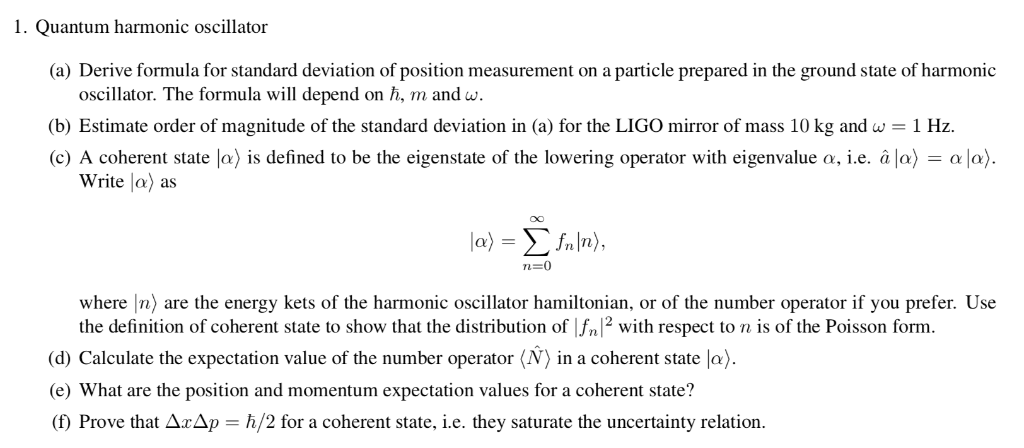1. Quantum harmonic oscillator (a) Derive formula for standard deviation of position measurement on a particle prepared in the ground state of harmonic oscillator. The formula will depend on h, m andw (b) Estimate order of magnitude of the standard deviation in (a) for the LIGO mirror of mass 10 kg and w 1 Hz. (c) A coherent state lo) is defined to be the eigenstate of the lowering operator with eigenvalue a, i.e. à lo)a) Write la) as where In) are the energy kets of the harmonic oscillator hamiltonian, or of the number operator if you prefer. Use the definition of coherent state to show that the distribution of Ifn12 with respect to n s of the Poisson form. d) Calculate the expectation value of the number operator (N) in a coherent state la) (e) What are the position and momentum expectation values for a coherent state? (f) Prove that Δ2Ap-h/2 for a coherent state, ie, they saturate the uncertainty relation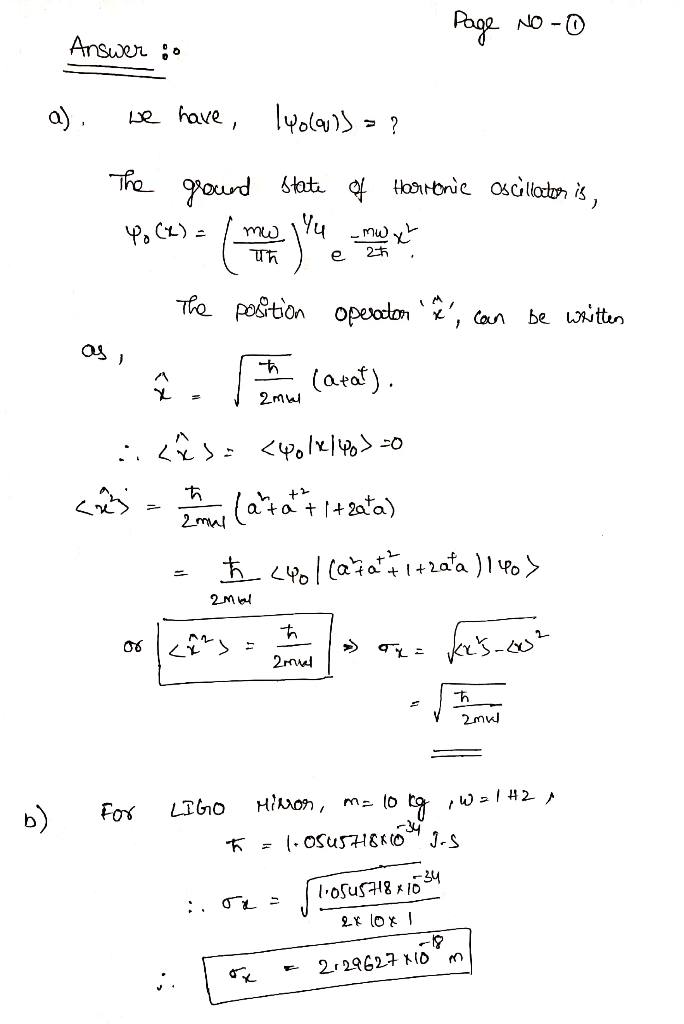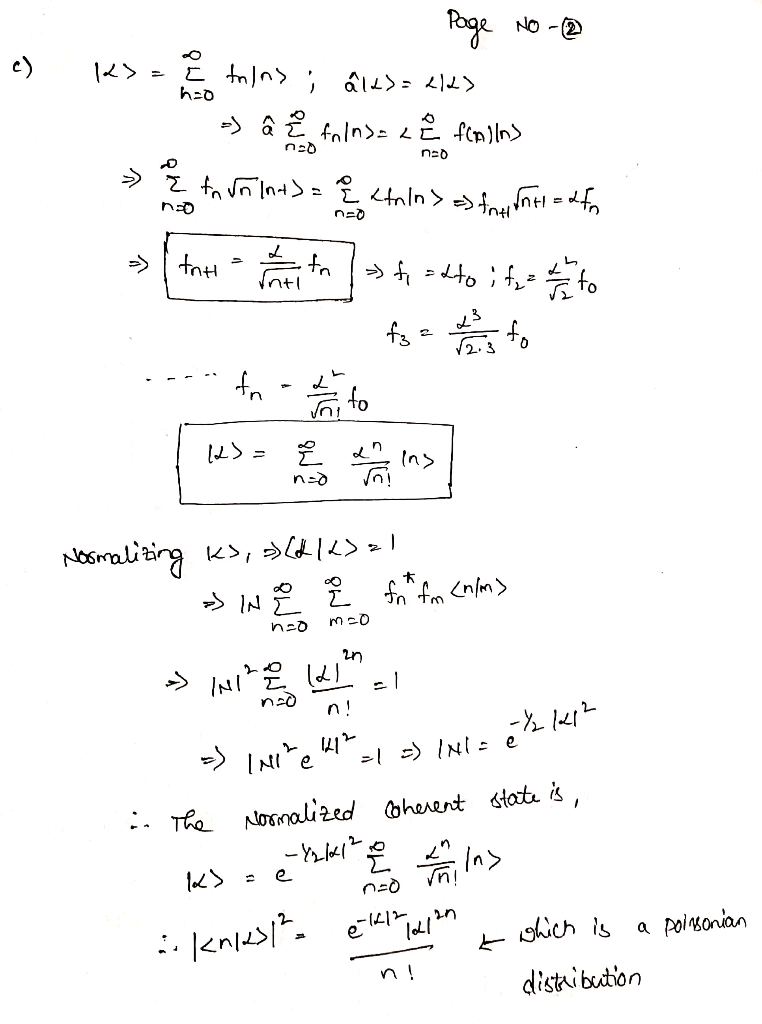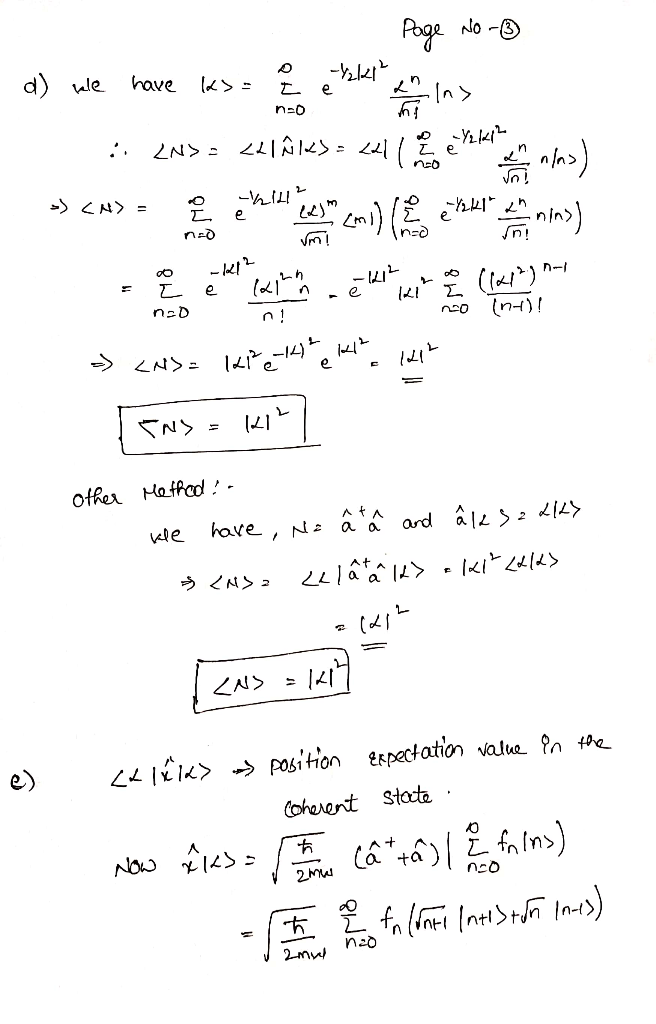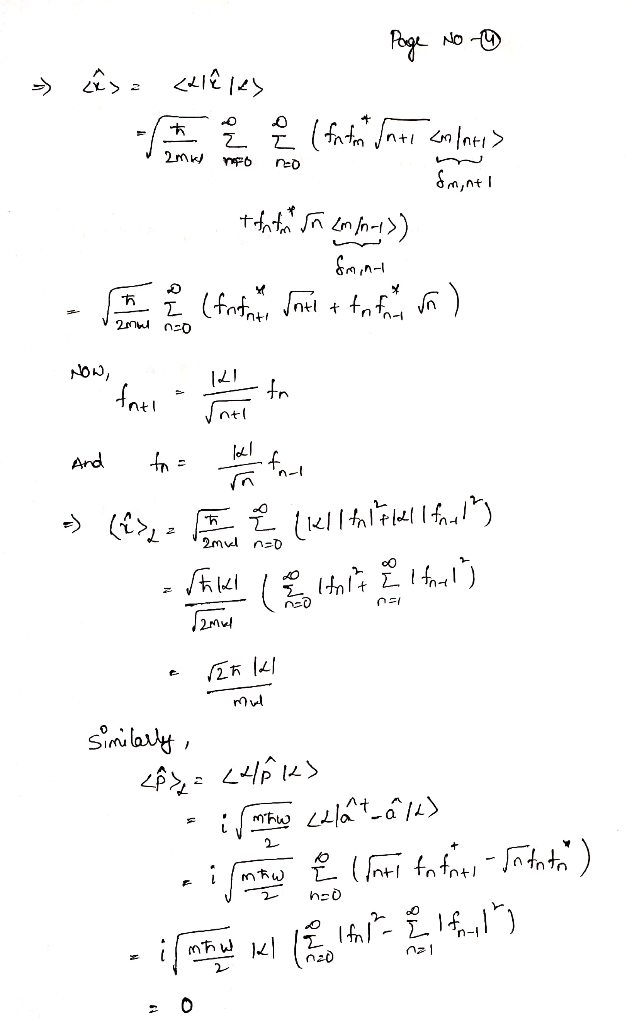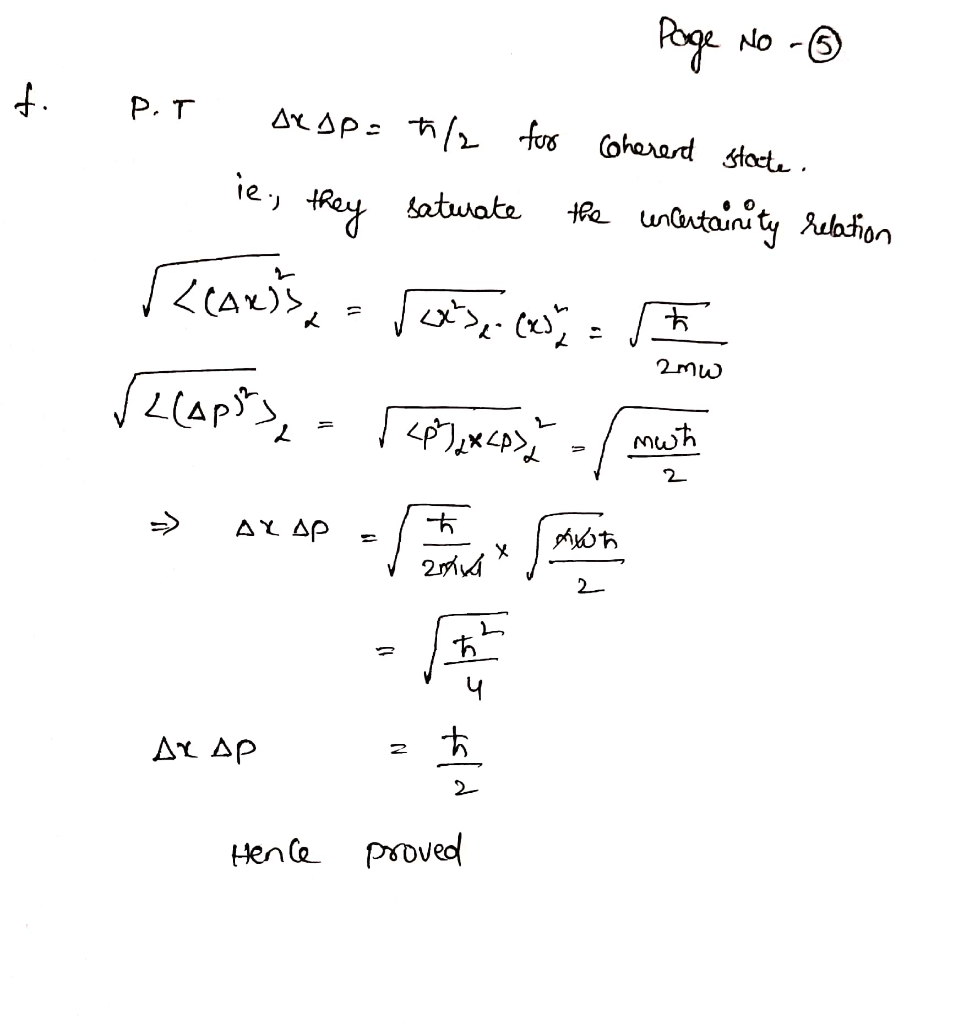Earn Coins

Coins can be redeemed for fabulous gifts.

Similar Homework Help Questions
• Harmonic Oscillator: Determine the expectation value of the position of a harmonic oscillator in its ground...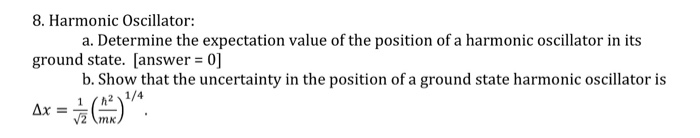Harmonic Oscillator: Determine the expectation value of the position of a harmonic oscillator in its ground state, Show that the uncertainty in the position of a ground state harmonic oscillator is Delta x 1/square root 2 (h^2/mk)^1/4.

• Intro to Quantum Mechanics problem: . In a harmonic oscillator a normalized "coherent" state ya(x) is...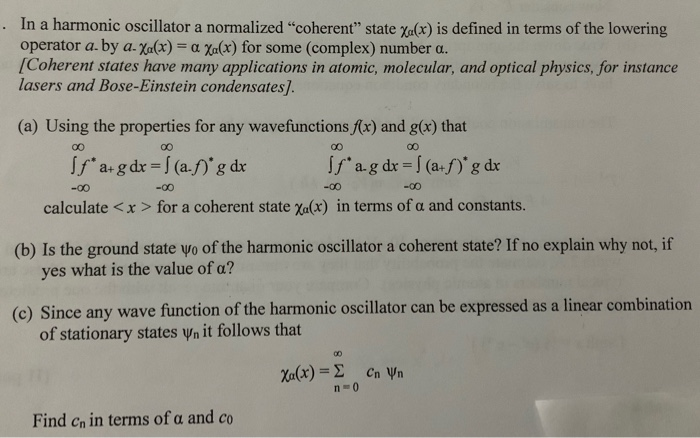Intro to Quantum Mechanics problem: . In a harmonic oscillator a normalized "coherent" state ya(x) is defined in terms of the lowering operator a. by aXa(x) = a Xa(x) for some (complex) number a. /Coherent states have many applications in atomic, molecular, and optical physics, for instance lasers and Bose-Einstein condensates]. (a) Using the properties for any wavefunctions f(x) and g(x) that 00 00 if ag dx (a.f)g dx f a+g dx (a.)'g dx -00 -00 -00 calculate <x >...

• In the lecture notes, we only solved the TISE for the quantum harmonic oscillator 1 Now,...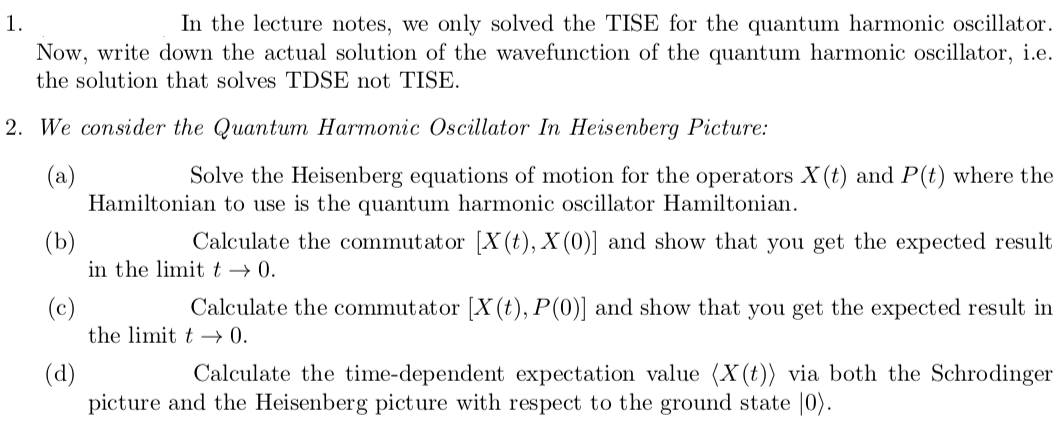In the lecture notes, we only solved the TISE for the quantum harmonic oscillator 1 Now, write down the actual solution of the wavefunction of the quantum harmonic oscillator, i.e. the solution that solves TDSE not TISE. 2. We consider the Quantum Harmonic Oscillator In Heisenberg Picture: (a) Hamiltonian to use is the quantum harmonic oscillator Hamiltonian Solve the Heisenberg equations of motion for the operators X (t) and P(t) where the Calculate the commutator [X(t), X (0)] and show...

• Question A2: Coherent states of the harmonic oscillator Consider a one-dimensional harmonic oscillator with the Hamiltonian...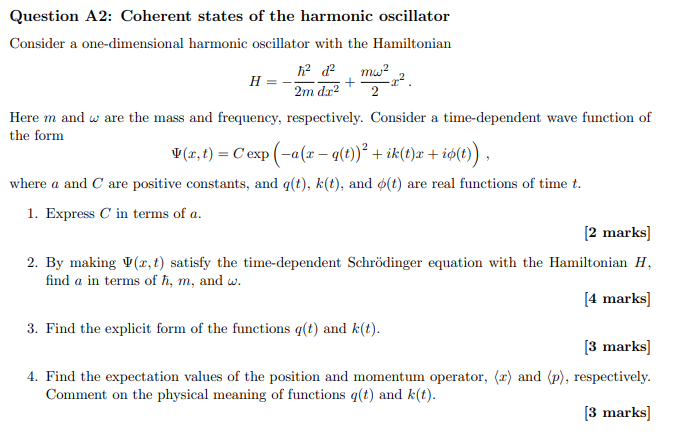Question A2: Coherent states of the harmonic oscillator Consider a one-dimensional harmonic oscillator with the Hamiltonian 12 12 m2 H = -2m d. 2+ 2 Here m and w are the mass and frequency, respectively. Consider a time-dependent wave function of the form <(x,t) = C'exp (-a(x – 9(t)+ ik(t)z +io(t)), where a and C are positive constants, and g(t), k(t), and o(t) are real functions of time t. 1. Express C in terms of a. [2 marks] 2. By...

• 5. Coherent States (Answer only question 5 for part a, b, and c) A coherent state is an Eigenstat...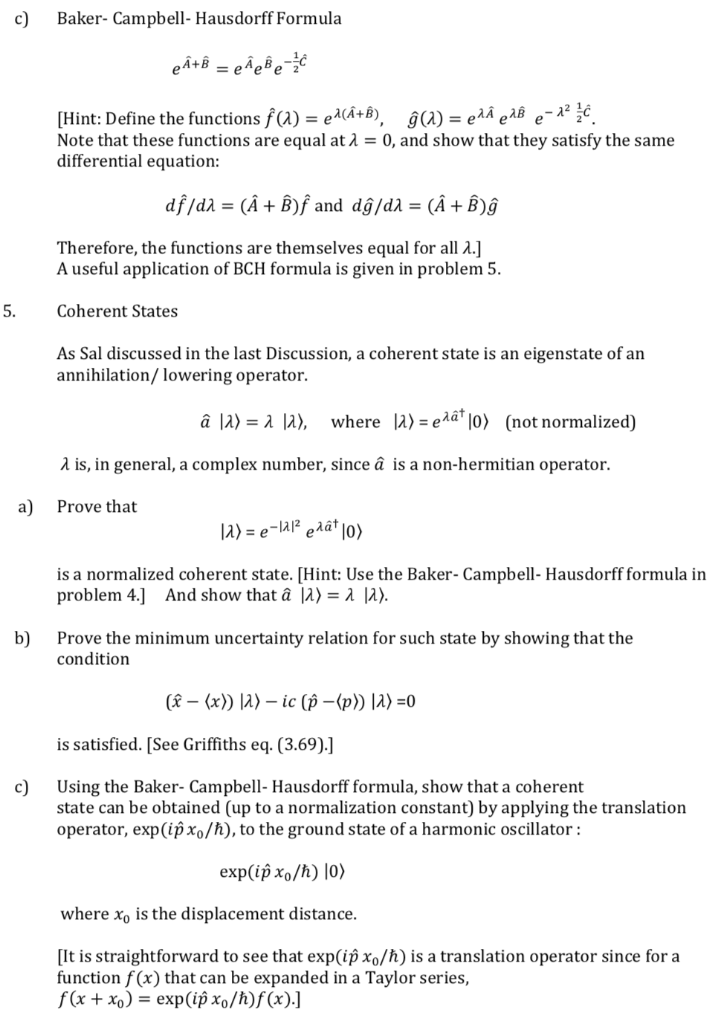5. Coherent States (Answer only question 5 for part a, b, and c) A coherent state is an Eigenstate of annihilation / lowering operator c) Baker- Campbell- Hausdorff Formula [Hint: Define the functions fa-eaA+8), ģ(A)-eä eABe-12 č. Note that these functions are equal at -0, and show that they satisfy the same differential equation: df/di (A+ B)f and dg/da (A+B)g Therefore, the functions are themselves equal for all λ.] A useful application of BCH formula is given in problem 5...

• Relevant information Need help with this Harmonic Oscillator question, thanks 11.5.3 Coherent states 1. Calculate (zPz)...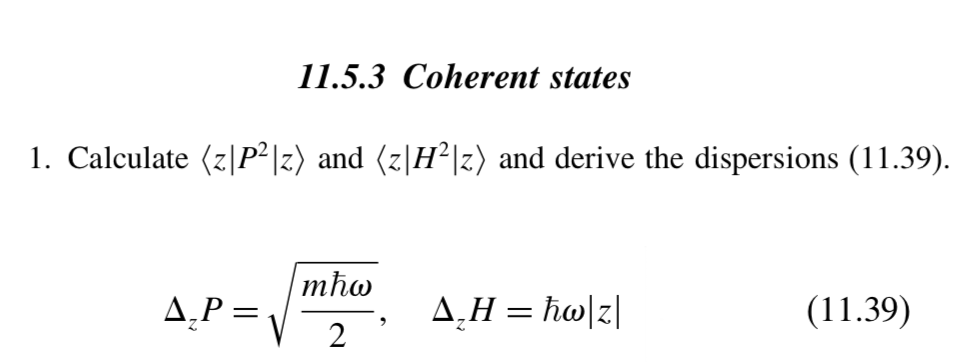Relevant information Need help with this Harmonic Oscillator question, thanks 11.5.3 Coherent states 1. Calculate (zPz) and (z|H2|z) and derive the dispersions (11.39) mha Д,Н 3 һо\2] Д.Р3, (11.39) 2 The coherent state z) is an eigenvector of the (non-Hermitian) annihilation operator a with eigenvalue (212 = (2 expectation values: /2hmw Im z, (z|P|z) 〈z|비) = hw( |zl2 A coherent state |z) is defined as n) e-l2/2ea'z]0) = e 7/M21- n=0 11.5.3 Coherent states 1. Calculate (zPz) and (z|H2|z) and...

• Please do this problem about quantum mechanic harmonic oscillator and show all your steps thank you. Q1. Consider a particle of mass m moving in a one-dimensional harmonic oscillator potential. 1....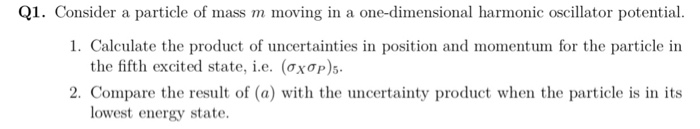Please do this problem about quantum mechanic harmonic oscillator and show all your steps thank you. Q1. Consider a particle of mass m moving in a one-dimensional harmonic oscillator potential. 1. Calculate the product of uncertainties in position and momentum for the particle in 2. Compare the result of (a) with the uncertainty product when the particle is in its the fifth excited state, ie. (OxơP)5. lowest energy state. Q1. Consider a particle of mass m moving in a one-dimensional...

• Problem #1 The explicit wavefunction for a particle in the n-1 state of the quantum harmonic...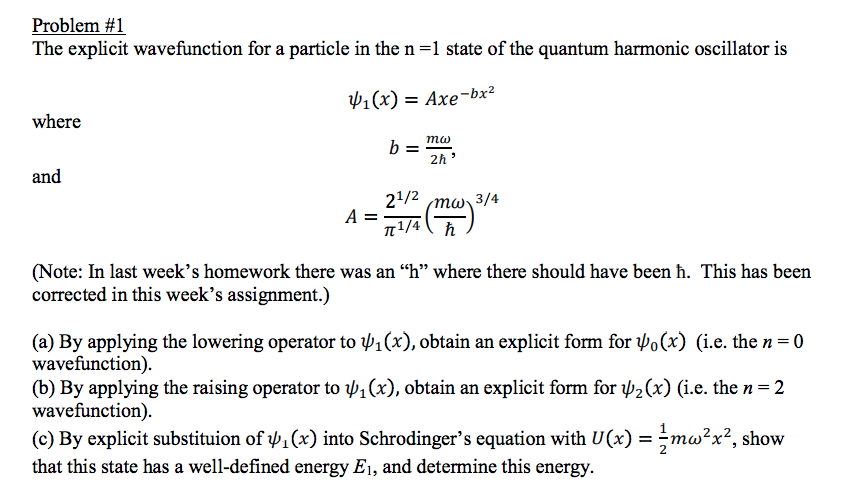Problem #1 The explicit wavefunction for a particle in the n-1 state of the quantum harmonic oscillator is p1(x)- Axe-bx2 where mo 2h and ?1/4 (Note: In last week's homework there was an "h" where there should have been ?. This has been corrected in this week's assignment.) (a) By applying the lowering operator to ),obtain an explicit form for o(x) (i.e. the n-0 wavefunction) (b) By applying the raising operator to x), obtain an explicit form for p2(x) (i.e....

• Problem 5. (30 points) Consider a Harmonic oscillator with H that H=(ata + 1 / 2)ho,...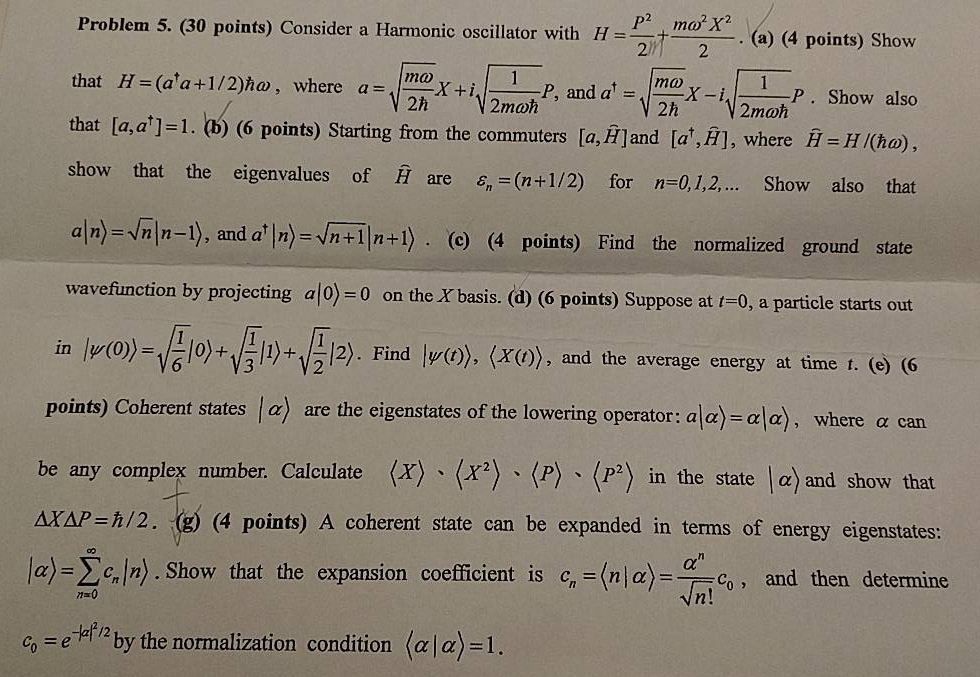Problem 5. (30 points) Consider a Harmonic oscillator with H that H=(ata + 1 / 2)ho, where a=dma)X + i (a) (4 points) Show P, and a x 2h 2h 2moh P. Show also 2moh that [a, a]-l. (b) (6 points) Starting from the commuters la, HJand la', A), where H-H(h) show that the eigenvalues of Hare e,=(n+1/2) for n-0, 1,2, Show also that alm)-nln-l), and a l). (( points) Find the normalized ground state wavefunction by projecting alo)-0 on...

• Quantum Chemistry. Thx in Advance! 1. For a harmonic oscillator with unit mass and unit frequency,...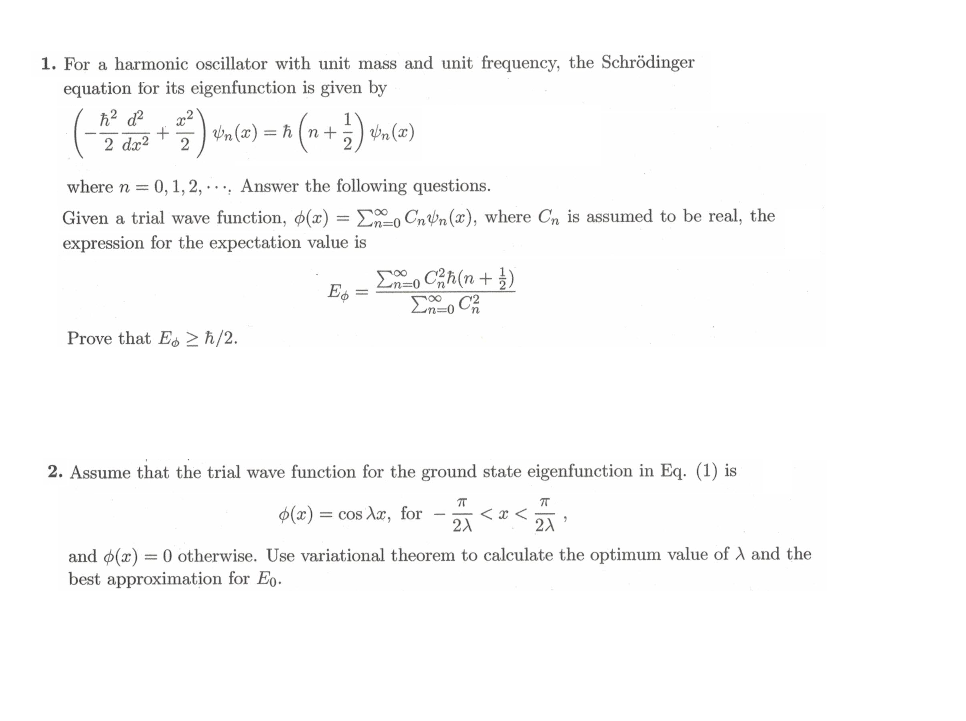Quantum Chemistry. Thx in Advance! 1. For a harmonic oscillator with unit mass and unit frequency, the Schrödinger equation for its eigenfunction is given by where n 0, 1, 2, . . .. Answer the following questions. Given a trial wave function, ?(x)-?000CnUn(x), where expression for the expectation value is is assumed to be real, the Prove that Eo2 h/2 2. Assume that the trial wave function for the ground state eigenfunction in Eq. (1) is ?(x) = cos Xx,...